Dave's Math Tables: Integral Identities(Math | Calculus | Integrals | Identities)

Formal Integral Definition: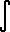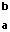f(x) dx = lim (d -> 0)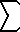(k=1..n) f(X(k)) (x(k) - x(k-1)) when...

a = x0 < x1 < x2 < ... < xn = b
d = max (x1-x0, x2-x1, ... , xn - x(n-1))
x(k-1) <= X(k) <= x(k)     k = 1, 2, ... , nF '(x) dx = F(b) - F(a) (Fundamental Theorem for integrals of derivatives)a f(x) dx = af(x) dx (if a is constant)f(x) + g(x) dx =f(x) dx +g(x) dxf(x) dx =f(x) dx | (a b)f(x) dx +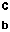f(x) dx =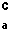f(x) dxf(u) du/dx dx =f(u) du (integration by substitution)